all flextable tags: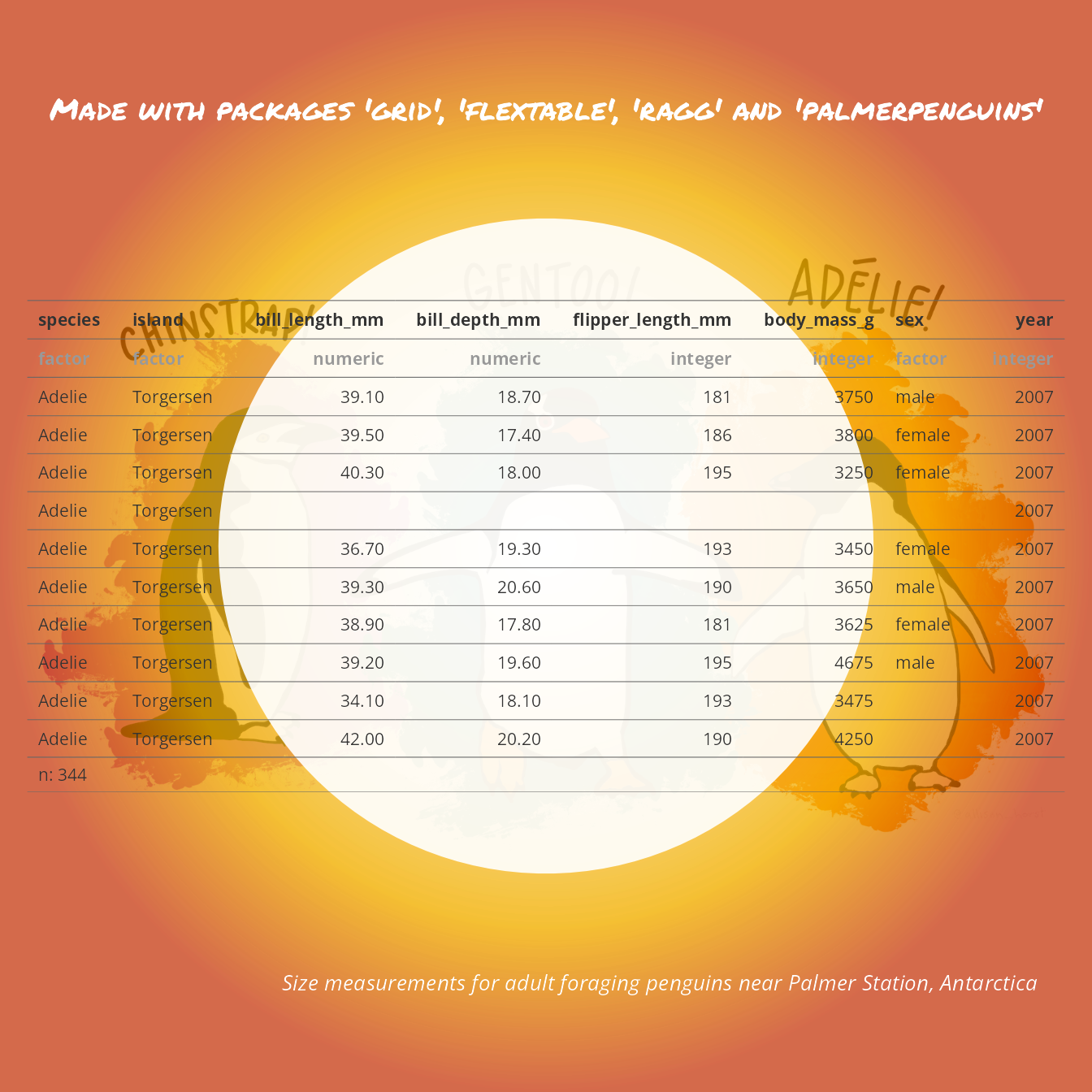#### grid clipping and flextable

An exemple that shows how to transform a flextable into a nice data-visualisation.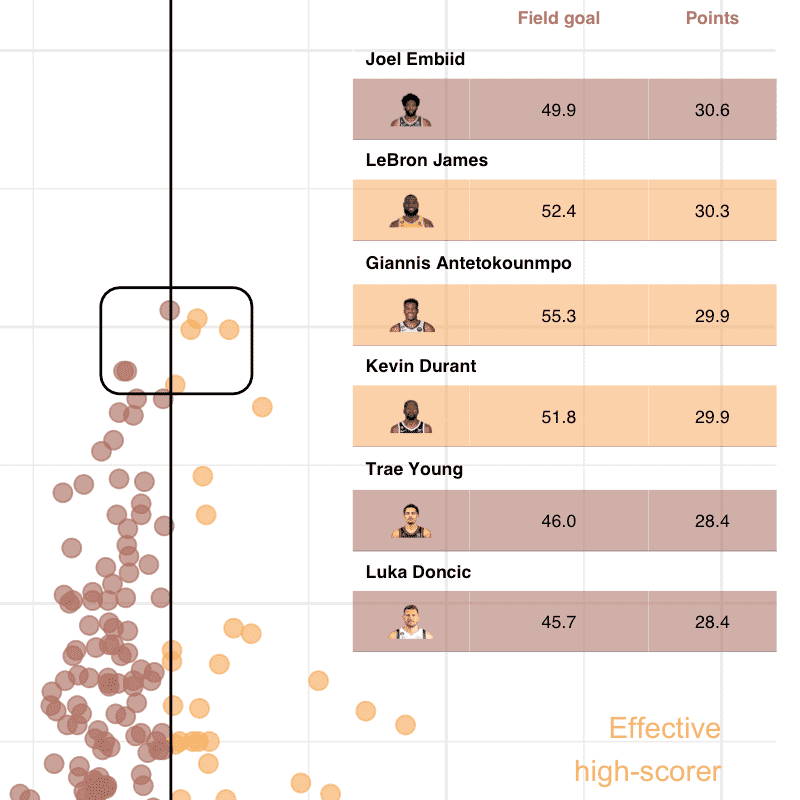#### Using flextable with ggplot2 and patchwork

Examples that show how to use flextable::gen_grob() and package ‘patchwork’ to mix graphics and tables.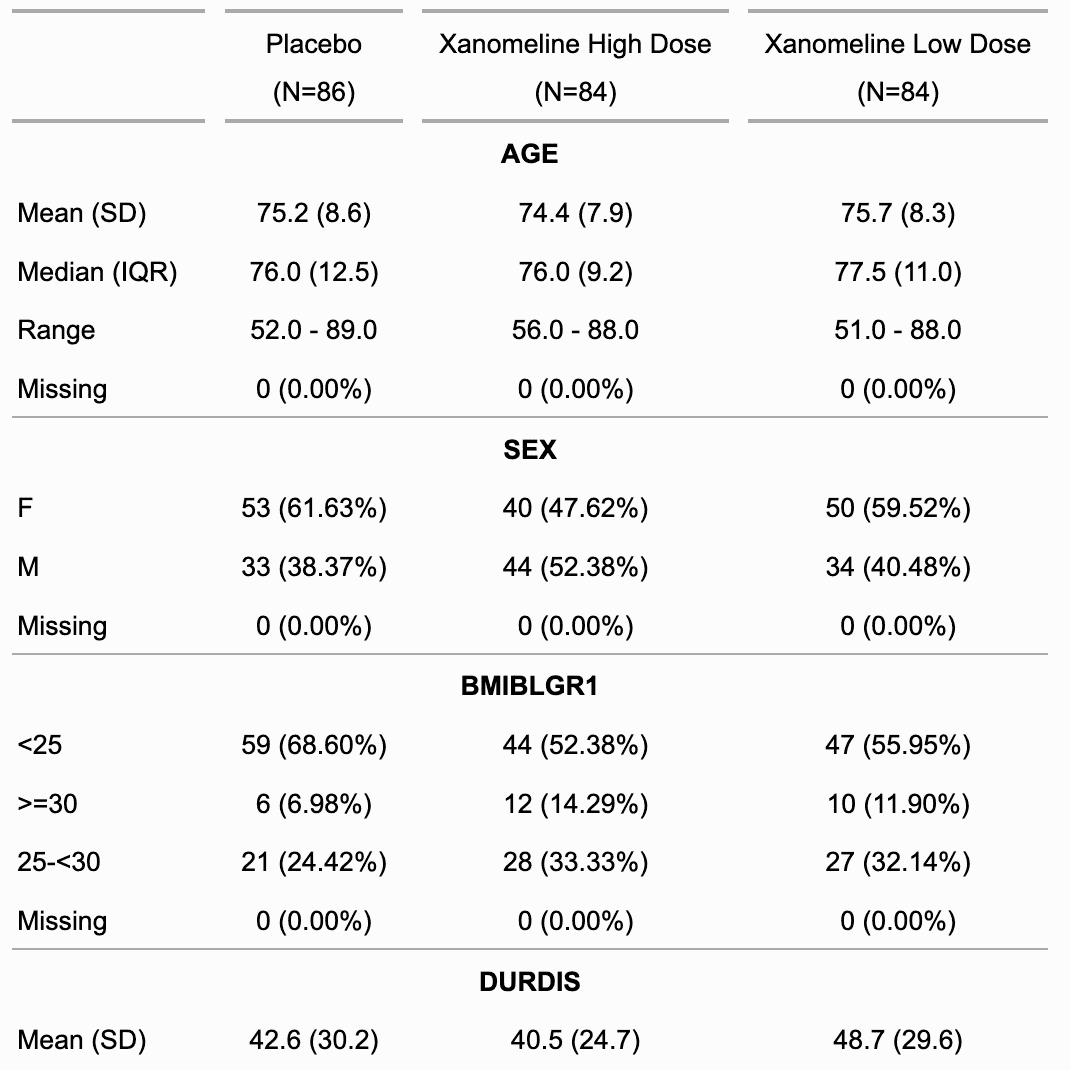#### Demographic Tables with flextable

This example shows how to use flextable to produce demographic tables using summarizor().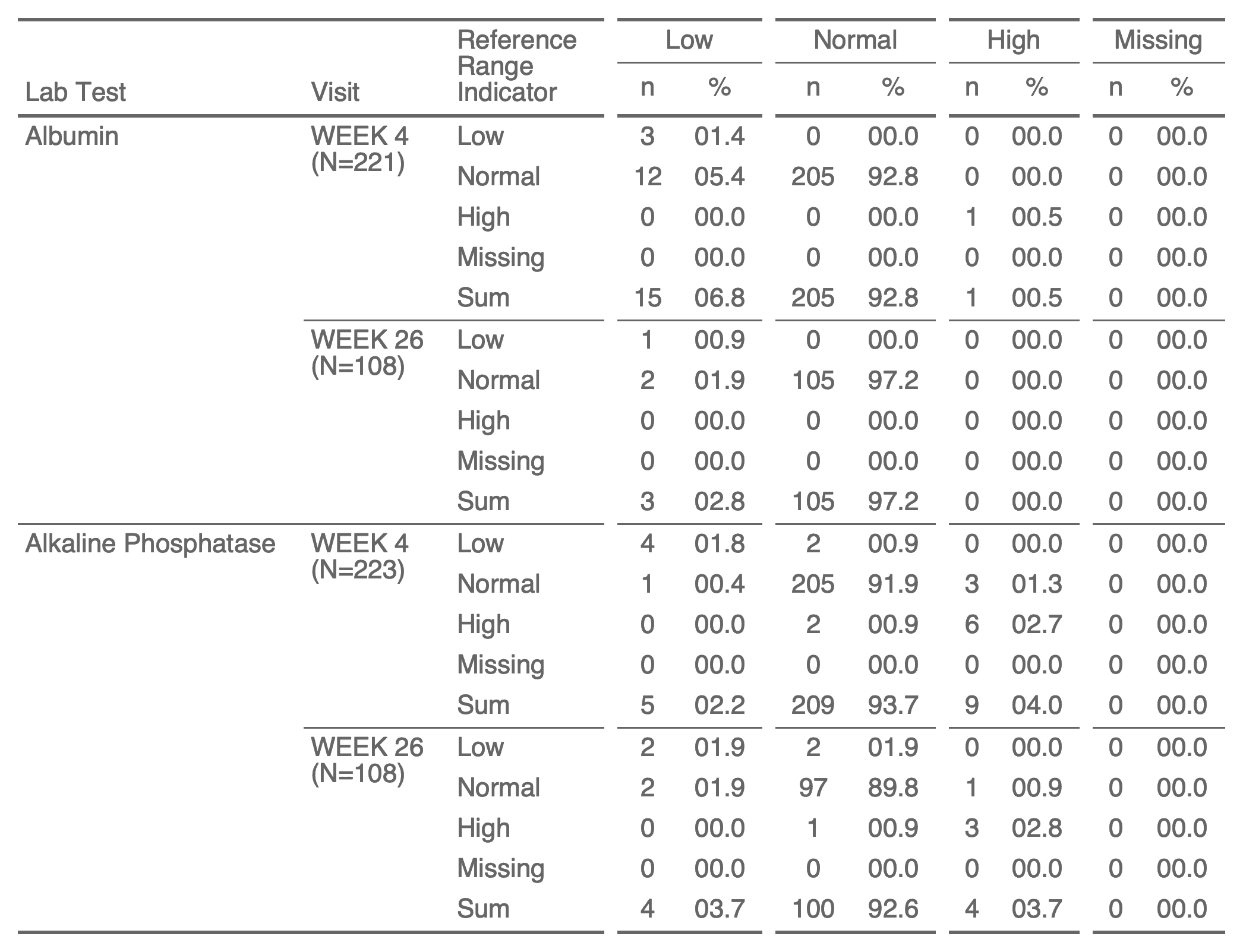#### Shift Tables with flextable

This example show how to use flextable to produce shift tables by using shift_table() and flextable::tabulator().#### Separate column names in header

This example show how to separate collapsed colnames into multiple rows with a dataset produced by dplyr::summarise().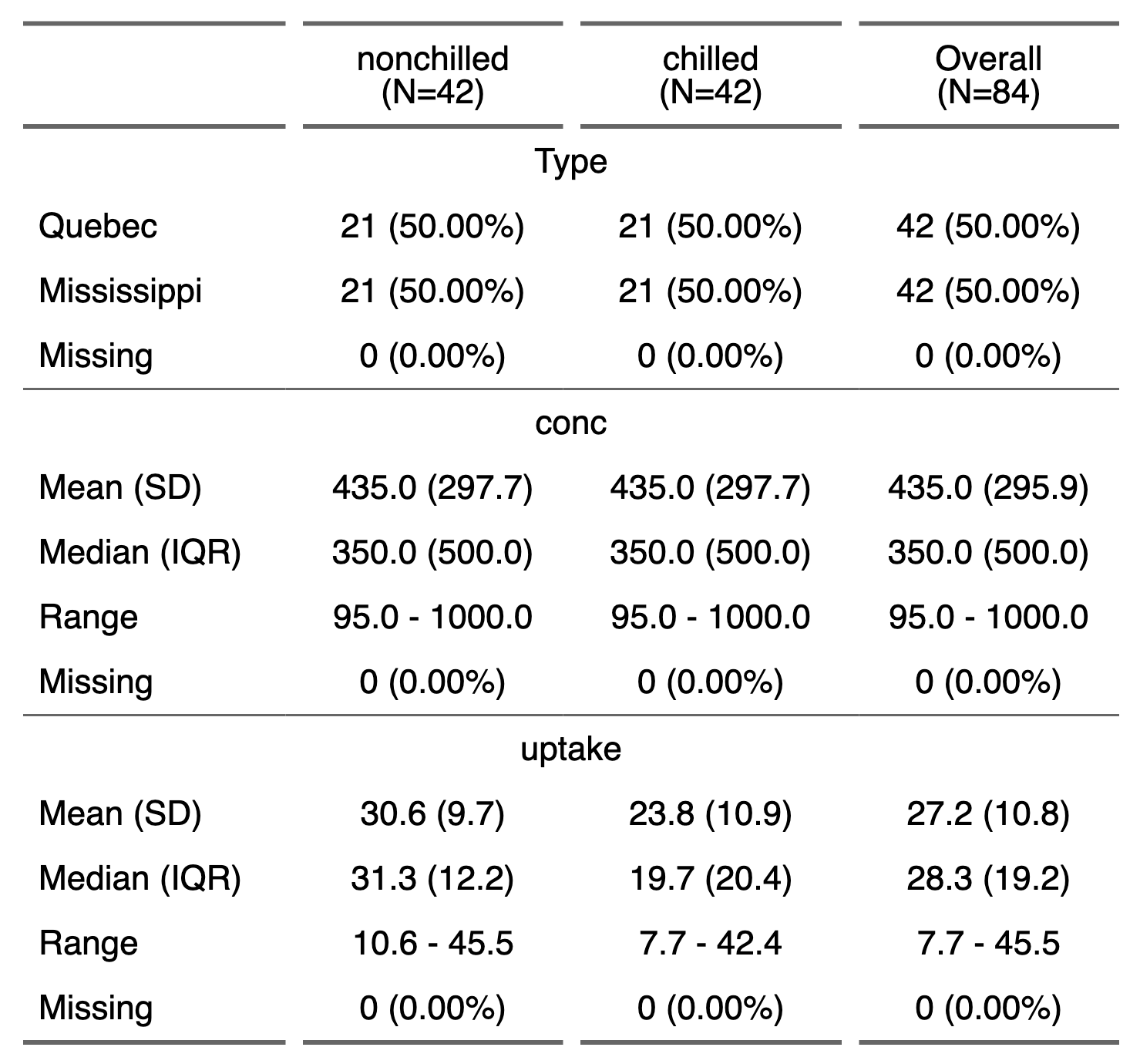#### Dataset summary with summarizor

This example show how to create a table presenting statistical summary of the columns of a data.frame with function summarizor().#### Nice cross-tabulated flextable

How to create a nice cross-tabulated flextable from aggregations. This example demonstrate how to use function tabulator().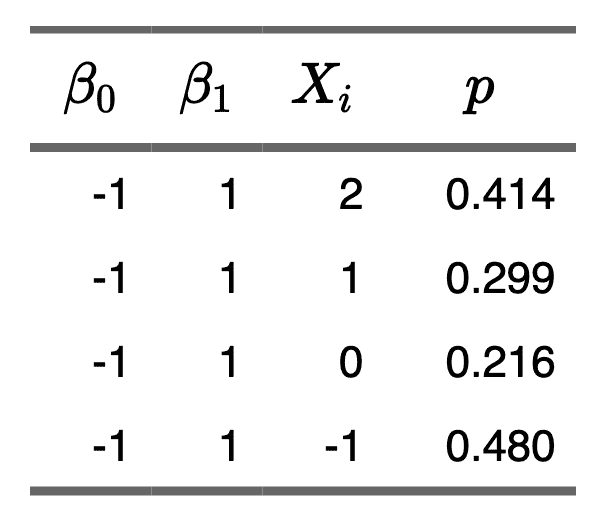#### Columns names with Katex

This example shows how to create a flextable equations in header by using LaTeX equations.#### Insert equations

This example shows how to add ‘Latex’ equations into a flextable.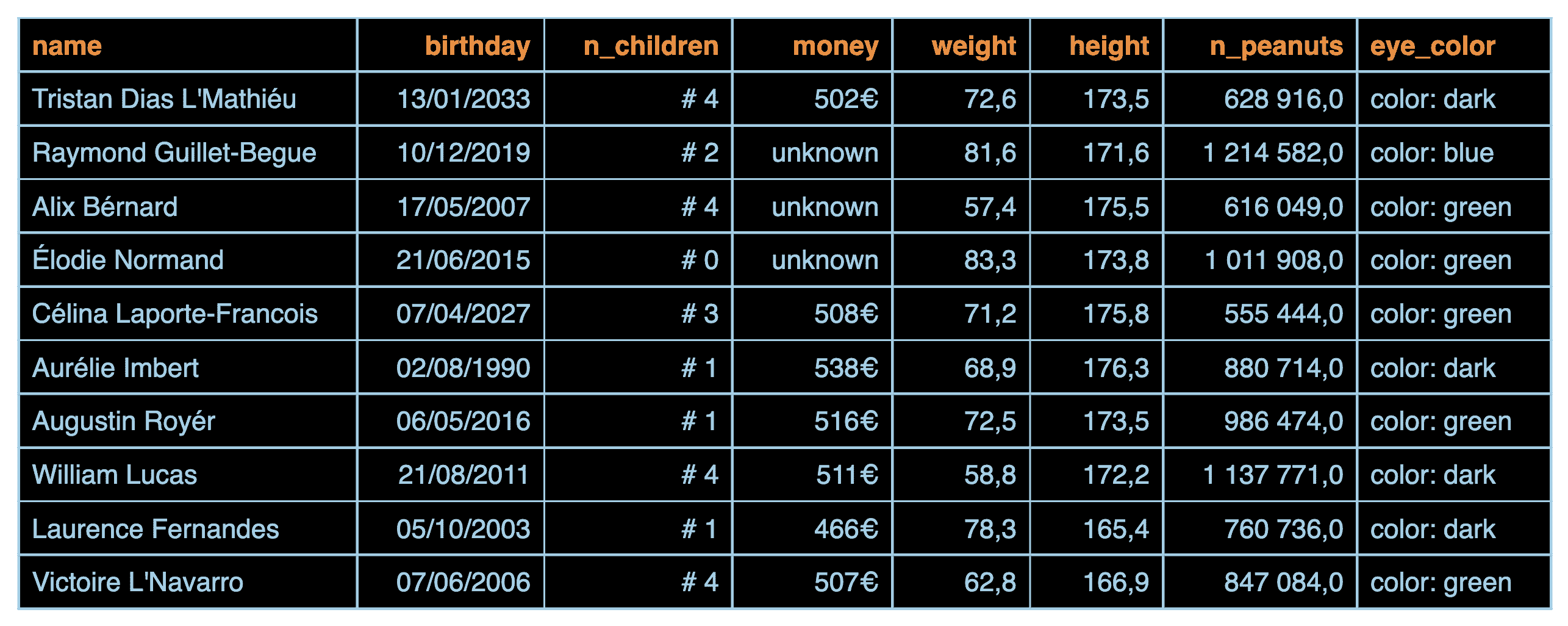#### Define how the text is displayed

Demonstration of formatting capabilities for cell content of various types such as numeric columns, dates, strings.Apply a color gradient on a contingency table.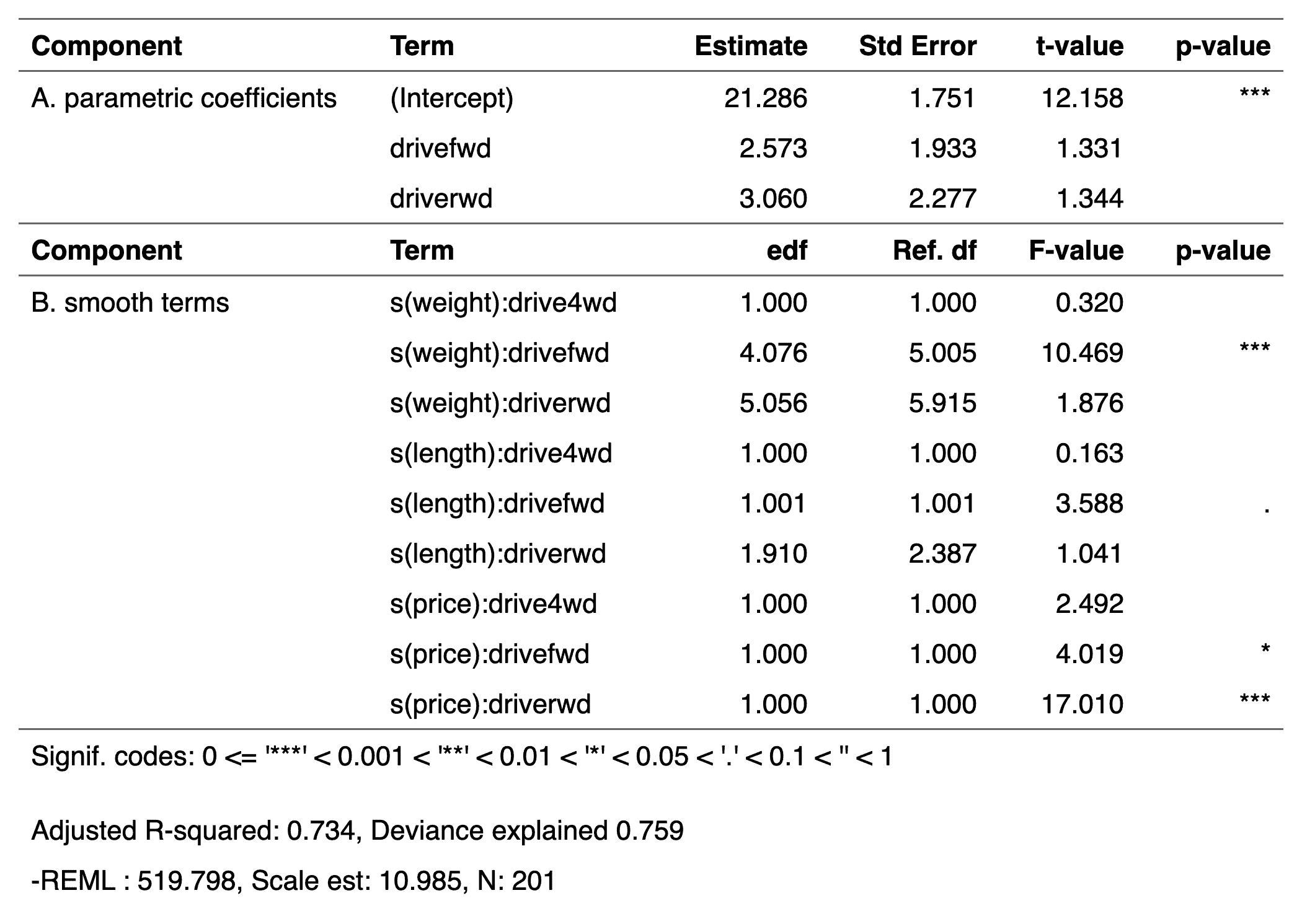#### GAM model

How to get a flextable from a GAM model by calling as_flextable.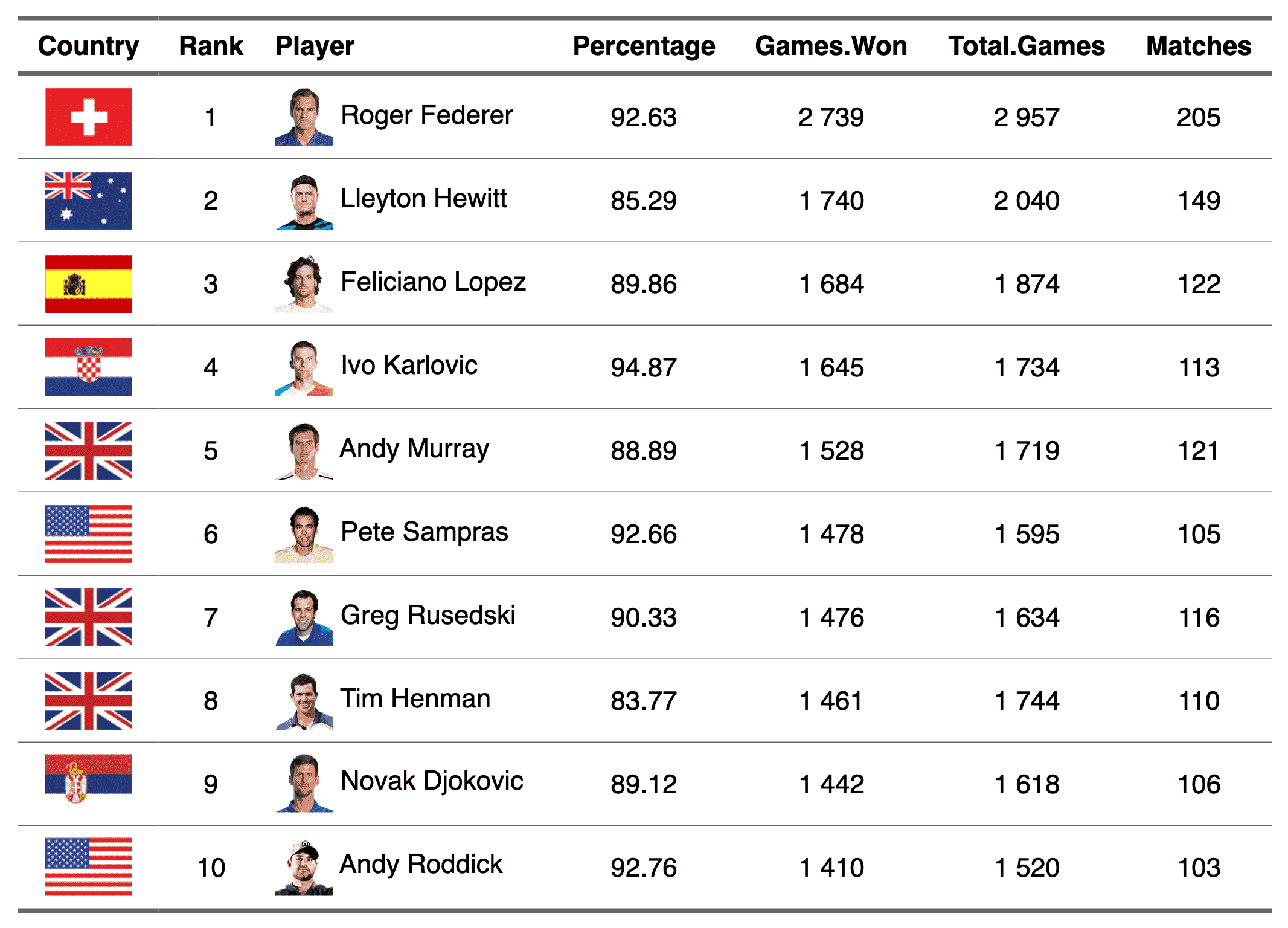#### Ranking of tennis players

Mix text and images inside a flextable with the functions compose and as_image.

#### captions examples

How to add captions to a flextable in R Markdown documents. These examples demonstrate various methods.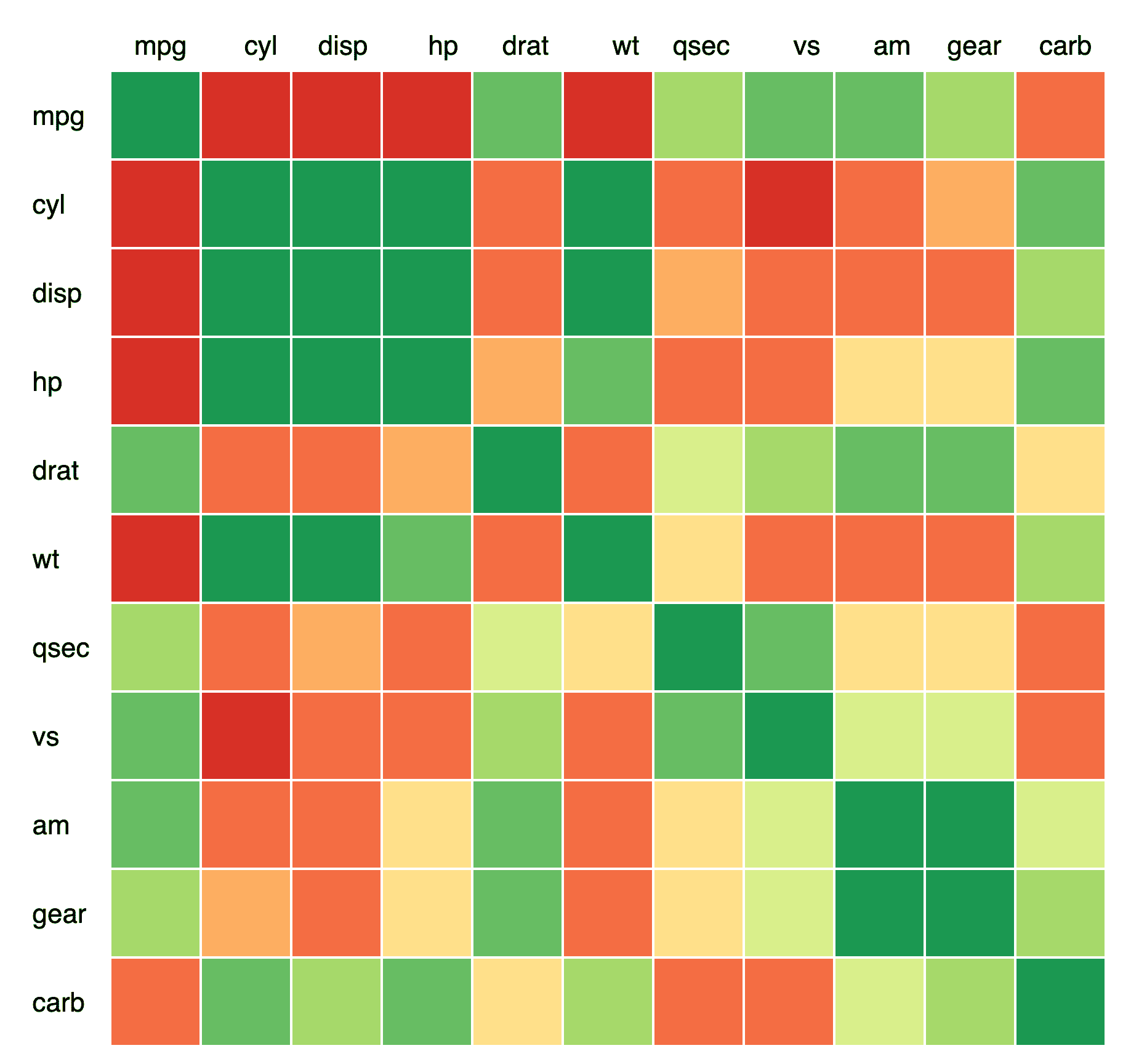#### Correlation matrix

How to add create a flextable that looks like an heatmap.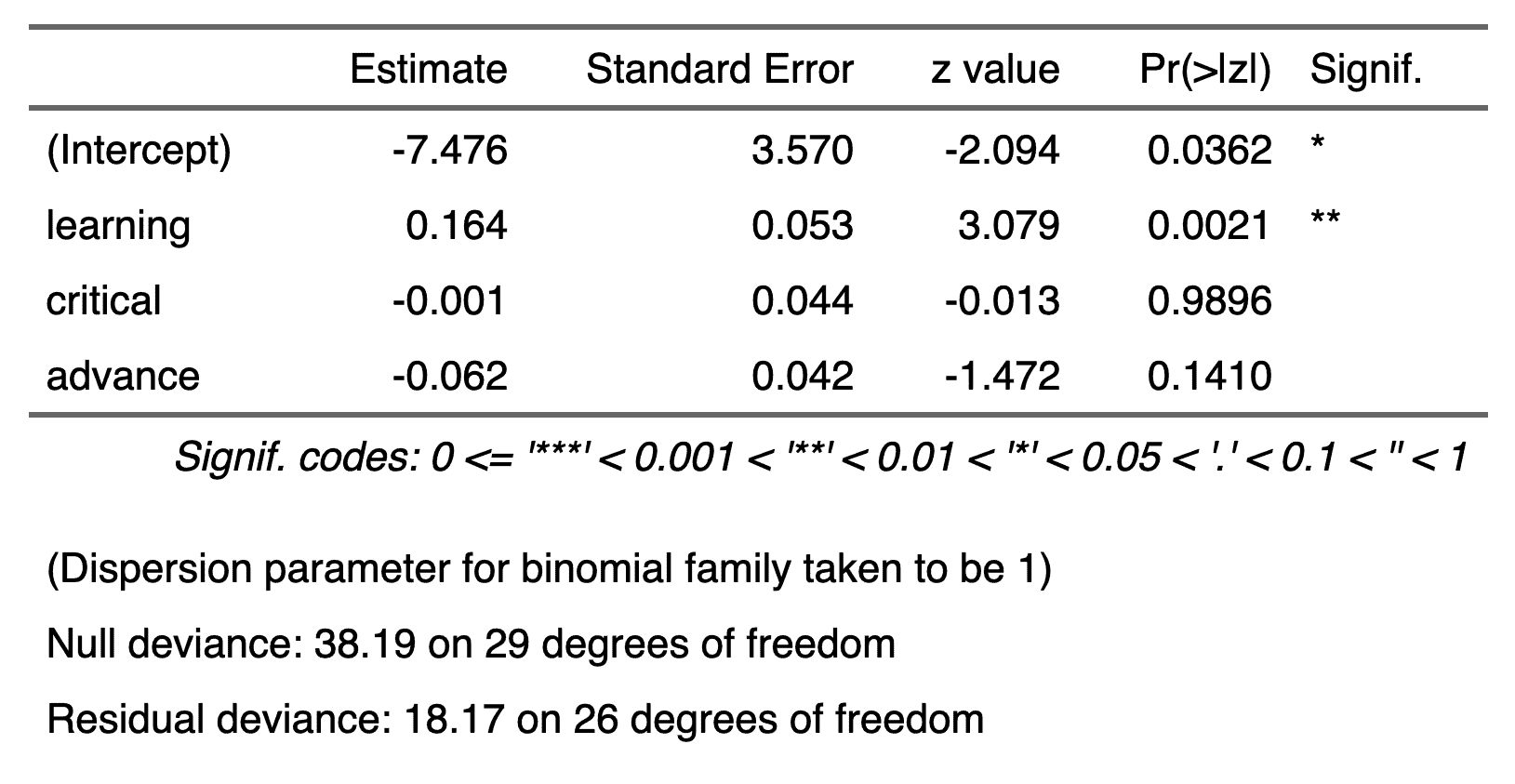#### GLM model

This example illustrates the creation of a flextable from a GLM model with a simple call to as_flextable.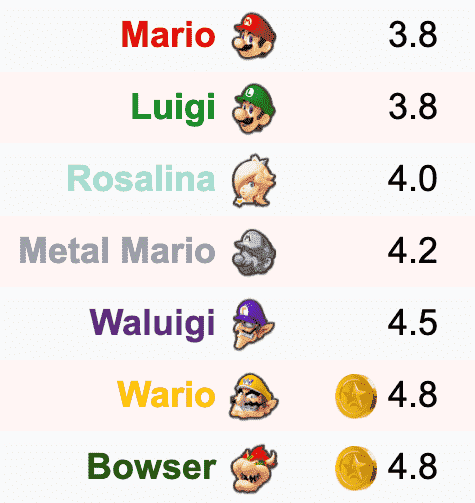#### Mario kart

Mix text and image to build a table presenting statistics of Mario Kart characters.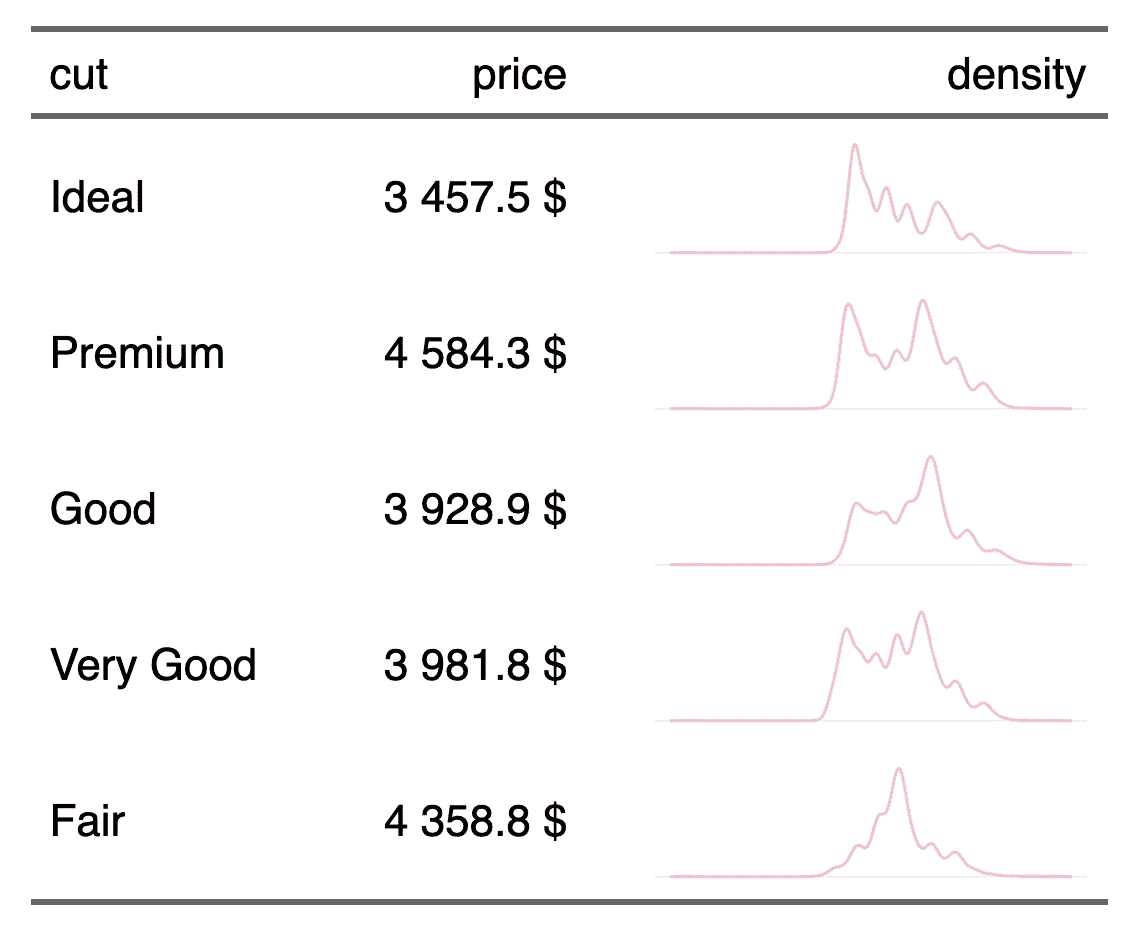#### Table with density lines

In this example, we create a flextable that represents aggregations and density curves together.#### Inner and outer borders

How to manage regular inner and outer borders.#### Creating a theme function

In this example, a theme function is created and used to format different data.frame.